*Penetration of Different Types of Radiation*
05-31-08

Summary: Radiation Penetration of gamma betas alphas and neutrons, why the penetrations look different.

The Exponential curve

Many people have heard of tenth value layers, and protection factors. Where does that come from?

A material as made up of a large number of atoms. Each atom in a material has a small area (cross-section) so radiation that travels through that material has some chance of striking the atom.

Here is a picture to illustrate some slab of material, and radiation traveling through it (figure 1). The radiation enters some slab of material, concrete or lead or even you, and that something is made out of lots of atoms, shown as green dots. Now in the first thin sliver of distance there are a few atoms, and the atoms are in the path of the radiation.  In a small sliver of thickness, there is also a lot of empty space. The chance of the radiation hitting an atom or just passing through is the ratio of the area of the atoms over the area of the slab. If we then looked at the next thin sliver behind the first one, there are some atoms, just like another figure 1, stacked behind the first layer. The chance that the radiation will hit some atoms or travel trough is again the ratio of the areas of the atoms to the area of the slab.

Adding more and more stacks of stuff leads to a constant chance of the radiation interacting in each thin slice. This means that in each thin slice, there is a constant fraction of the radiation removed, which means that after a distance in the material,

the amount removed is (fraction) x (fraction) x (fraction) x (fraction)…or some amount  that depends on the radiation and the material raised to the power of the number of thin slices that the radiation travels through. Because of this, the radiation would decrease as a constant exponential curve.Figure 1 Radiation enters a slab of something (concrete, lead, water, maybe even you) and that something has lots of tiny atoms in it (the green dots). After moving through a small thickness the chance that the radiation has hit something is the ratio of the area of all the atoms in that small slab, divided by the total area.

Gammas, X-rays and Neutrons

X ray radiation or gamma rays are high energy light rays, and at high enough energies act more or less like little particles.  Neutrons are heavy particles found in the nucleus of an atom, and have no electric charge. Because gamma rays x-rays and neutrons have no charge they interact infrequently with the materials they pass through, and so the exponential curve describes their penetration quite well. In other words, thinking of an x-ray or neutron as either doing nothing or hitting a material is a good approximation.

Figure 2 shows a picture of how gamma rays penetrate through a material. They are described by an exponential curve very well. The small buildup in radiation near the surface is only every seen in very high quality radiation that has all of the low energy stuff cleaned out. The example shown in figure 2 is actually from a medical accelerator, and reasonable care is taken to make sure the beam is quite “hard” (high energy) so the skin can be spared a lot of undesirable dose.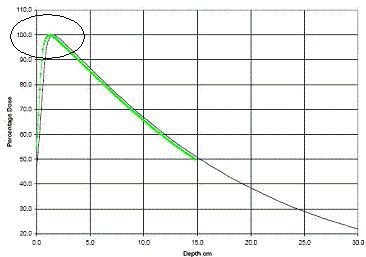Figure 2 Penetration of a very high energy beam of gamma rays in water. The curve follows an exponential very well. The circled area near the top left of the image shows the effect of the electrons in  the water being “kicked” down stream by the gamma rays (towards the right in this image). This effect will only ever be seen in beams without much low energy radiation, i.e. a medical radiotherapy machine or a high energy physics lab.

Electrons

Beta particles are high energy electrons and are given off by many radioactive materials. Since an electron is very light relative to an atom (about 2000 times less massive than a Hydrogen atom) electrons tend to scatter and bounce around a lot when they hit materials. Electrons are also charged particles, and this charge is always affecting everything around them. The charge of the beta particle will continuously cause ionization along the path that it is traveling.

Because of this, when a beta particle enters some material, it begins slowing down, and slows down continuously. The continuous slowing down is referred to, very ingeniously, as the “Continuous Slowing Down Approximation” or CSDA. For a high electron the energy loss per unit length is about 2MeV per centimeter in water. So, a 2MeV beta particle after traveling 0.5 cm in water will have energy of approximately 1 MeV. (2MeV x 0.5cm = 1MeV lost, and 1MeV remaining).

So there is continuous slowing down and bouncing around of a beta particle as it travels through a material. A good rule of thumb (i.e. one used every day treating patients for radiation) is to multiply the energy loss buy the density of material. So, if an electron is traveling through lead, which has a density of 11.2 times that of water, the energy loss is 11.2 x 2 = 22.4 MeV per cm. For high atomic number materials, there is a chance of the beta particles loosing energy by producing some x-rays. In water, the x-ray production from a beta particle is quite miniscule.

[The mathematical reader might notice that a change in energy (E) per change in length (x) can be written as dE/dx, a quantity referred to as the “stopping Power” of a material.]

What does this mean for the penetration of beta particles? In figure 2, the penetration of a  high energy electrons is displayed (in fact much higher energy than most beta particles) A beam of electrons, all of the same energy will continuously slow down and there will be none left after a distinct, exact distance. In the medical field, the distance is called the practical range and usually denoted by Rp. Again the electrons are entering from the left, and there is this “buildup effect” because this is a very high quality medical electron beam.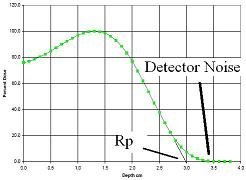Figure 3 Radiation dose as a function of depth for a high energy beam of electrons. In this case the electrons all start with the same energy. The electrons ionize the material they are in, loose energy and stop after a specific distance. This is referred to as the Practical range (Rp).

Electron emitters like Strontium 90 or Phosphorus 32 emit electrons with a broad range of energies. This means the penetration of the electrons is a jumble of different electron curves, all of which would individually look like the curve in figure 3. The penetration of electrons from real Strontium 90 and sources are shown in figure 4. In practice it is very difficult to measure the dose near the surface of material (special extrapolation chambers do exist for this). The images from figure 4 are from [Tandon 2006, Reft 1990].

To convert these curves for another material, the simple approximation is to reduce the range by the relative density of that material.  For example, acrylic has a density of about 1.15 times that of water, so the range in acrylic for the electrons is 1/1.15 less. Aluminum has a density of about 2.7 that of water, so an electron beam that can travel 1 cm in water, has a range of about 1/2.7 =  0.37 cm in Aluminum. As an example, 32P has a maximum beta energy of about 1.7MeV, and an average energy of about 0.7 MeV, so the average range of 32P would be about 07/2 = 0.35 cm (about an 1/8th of an inch of water), with nothing after about 3/8th of an inch.

At first glance, it might be thought that the curves in figure 4 look like exponential curves. However, we know that electrons are loosing energy continuously, so after a specific distance, there is no radiation. For gamma rays, the radiation is continuously attenuated so there will always be a small fraction passed through any barrier. That fraction might be very small however.  Because all of the electron energy will disappear after a predictable distance, the idea of a half value layer for electrons is bogus, even though some references will quote it.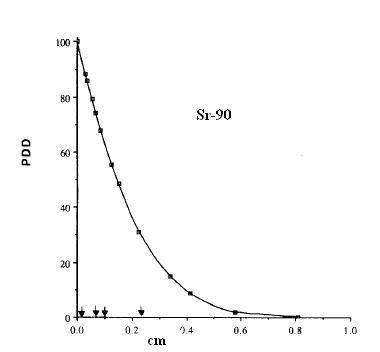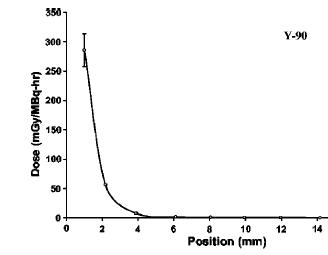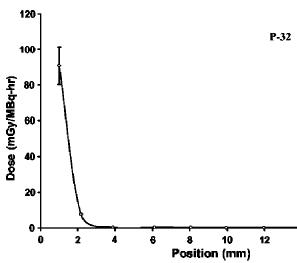Figure 4 The penetration of electrons in water from Strontium 90, Yttrium 90 and Phosphorus 32. The 90Sr value is from a large Opthalmic applicator, and the 32P and 90Y are for small point sources. These curves are for descriptive purposes, and will vary with the shape of the source. These curves represent a mixture of different electron energies, and each specific energy would have a penetration looking like figure 3.

Alpha Particles and Heavy Nuclei

Alpha particles are helium nuclei and are about 8,000 times heavier than electrons. Because of their mass when alphas travel along they smash their way through everything. Because they are so much heavier than electrons, they do not loose much energy when they hit an electron. Think of this like a SUV (8,000lbs) hitting a baseball (1 lb). The SUV isn't going to notice the baseball hardly at all. Like electrons alpha particles, protons, and heavy nuclei are continuously loosing energy. However they are loosing it in a slightly different way, and the rate that they loose energy is roughly inversely proportional to the amount of energy that they have. So an alpha particle with a high energy is slowly loosing energy while traveling through a material (but still loosing energy). Once it looses some energy, it has less energy, so it looses energy faster, and then a little faster, and finally when it has hardly any energy left, it looses it all very rapidly. This means that the radiation penetration of heavy particles like alpha particles looks different from electrons and gamma rays. An example of a proton ration dose is shown in figure 5 from [AAPM 2002] [Bragg Peak]. The penetration of an alpha particle or heavy ion with a distinct energy will look very similar to figure 5.

Again in practice the alpha particles emitted from a real nucleus will be a jumbled up range of energies, so the radiation penetration will be spread out. A realistic alpha particle penetration is shown in figure 6.

Similarly to electrons, the protons, alpha particles and heavy particles will continuously loose energy, and after a specific distance there will be no radiation left. The only detectable amount of radiation will be a small amount of x-ray’s (and a few neutrons) produced by the particles in the material, and mostly this will be miniscule for a low atomic number material like water. The range of typical alphas is very small [Triumph 1996] and is around 4 inches of air for the alphas from many radioactive nuclei. Dividing 4 inches by the relative density of water to air (about a thousand) gives a range of an alpha at thousandths of an inch in water. In metric terms, 100mm divided by a thousand is 0.1mm of water, which is a quite good estimate of the ranges of alphas in water.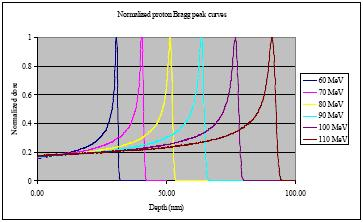Figure 5 An example of the penetration of very high energy protons through water. These are the results that might be seen for very pure laboratory beams with a specific energy. Compare this the the x-rays from figure 2.

Lower energy protons will produce very similar distributions, with the depth of penetration reduced. The radiation penetration of protons or alpha particles from a real isotope will be a combination of many of these curves, all of different energies, smoothing out the shape of the curve.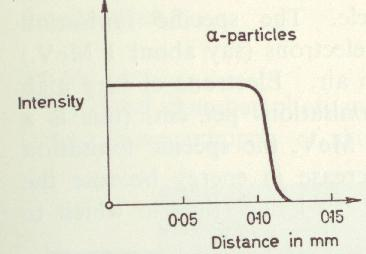Figure 6Example of penetration of alpha particles in water for a typical isotope. Since a radioactive source produces a whole range of energies of alphas, all of the nice curves from figure 5 are squashed together to give the shape shown. From [Young 1967].

Again, with the heavy ions and protons, there will be a specific distance after which there is no more radiation. There is no half value layer, or signal in micro rads or nano rads that can be detected. This means that without a special detector with a thin window or no window detecting alphas is a non-starter. With gammas, a detector can be across the room, or across the road and if it is sensitive enough it can detect a signal. With alphas, no matter how big the source is, there will be no signal.

[As a note: If there is any radiation after the practical range it is the miniscule amount of contamination in the beam. With heavy ions or protons in water, the amount of x-rays produced is very small. Compared with electrons, the protons and heavy ions are very heavy, so they decelerate much more slowly when they interact with an atom. Because of that, the Bremsstrahlung “breaking radiation” is corresponding much smaller.]

Summary

Gamma rays, x-rays, and neutrons, being neutral will only lose energy when they hit something (like an atom).Because of this, Gamma rays will loose a constant fraction of energy for each unit of distance, so the amount of radiation will be (some fraction) x (some fraction) x (some fraction) …after traveling a distance. There will always be a small amount, but it can be reduced until it is comparable with the background.

Electrons, Protons, Heavy ions and Alpha particles loose energy continuously because they are charged and are continuously interacting. Given the initial energy of the particle, the exact final point were the particles slow down and stop can be determined.Unlike the gamma arrays, there is nothing after that point, unless it is a miniscule contamination of x-rays produced in the material.

References

[Welsh 2006] Welsh, JS “Beta Radiation”. The Oncologist (11) pages 181-183 2006

http://theoncologist.alphamedpress.org/cgi/reprint/11/2/181

[Bragg Peak] http://en.wikipedia.org/wiki/Bragg_Peak

Offline references

[Tandon 2006] Tandon, P. “Estimation of radiation dose at various depths for commonly used radionuclides in radiosynoviorthesis in a tissue equivalent material”. Medical Physics (33) 2744 2750

[Reft 1990] Reft, C. “Dosimetry of Sr-90 opthalmic applicators”. Medical Physics (17) pages 641-646 1990

[Young 1967]  Young MEJ Radiological Physics 2nd Ed. Charles Thomas 1967.